Learn all Concepts of Chapter 5 Class 10 (with VIDEOS). Check - Arithmetic Progressions - Class 10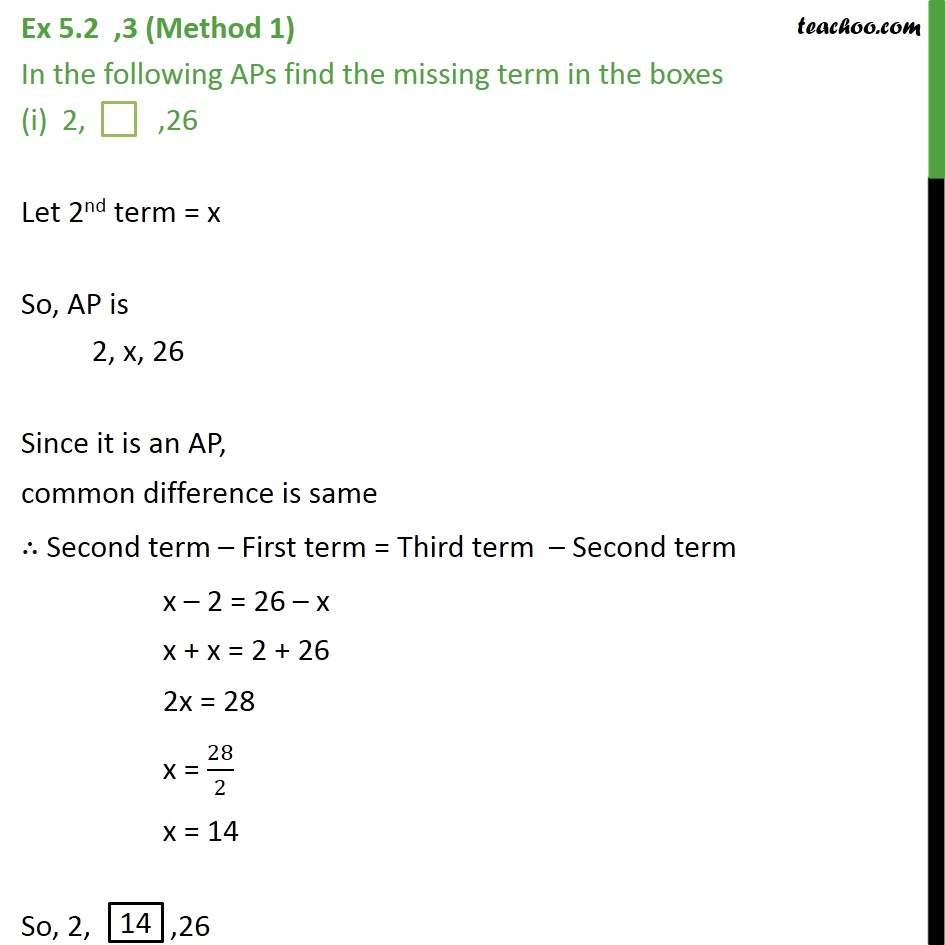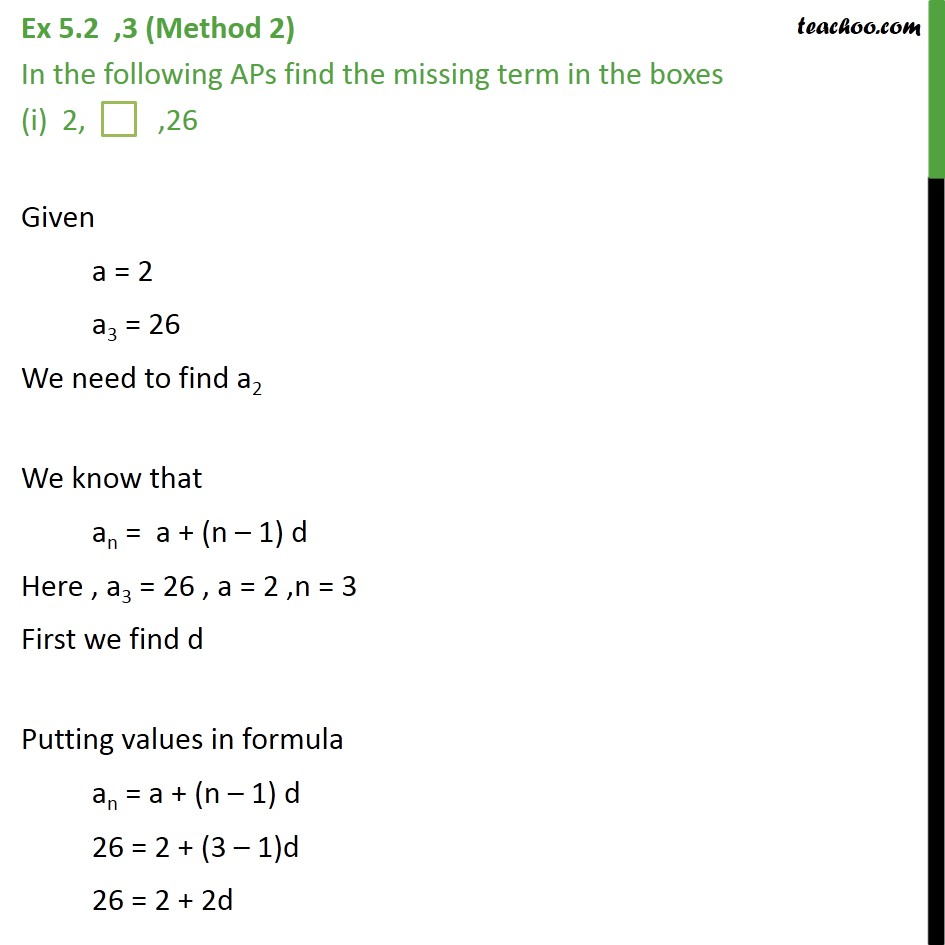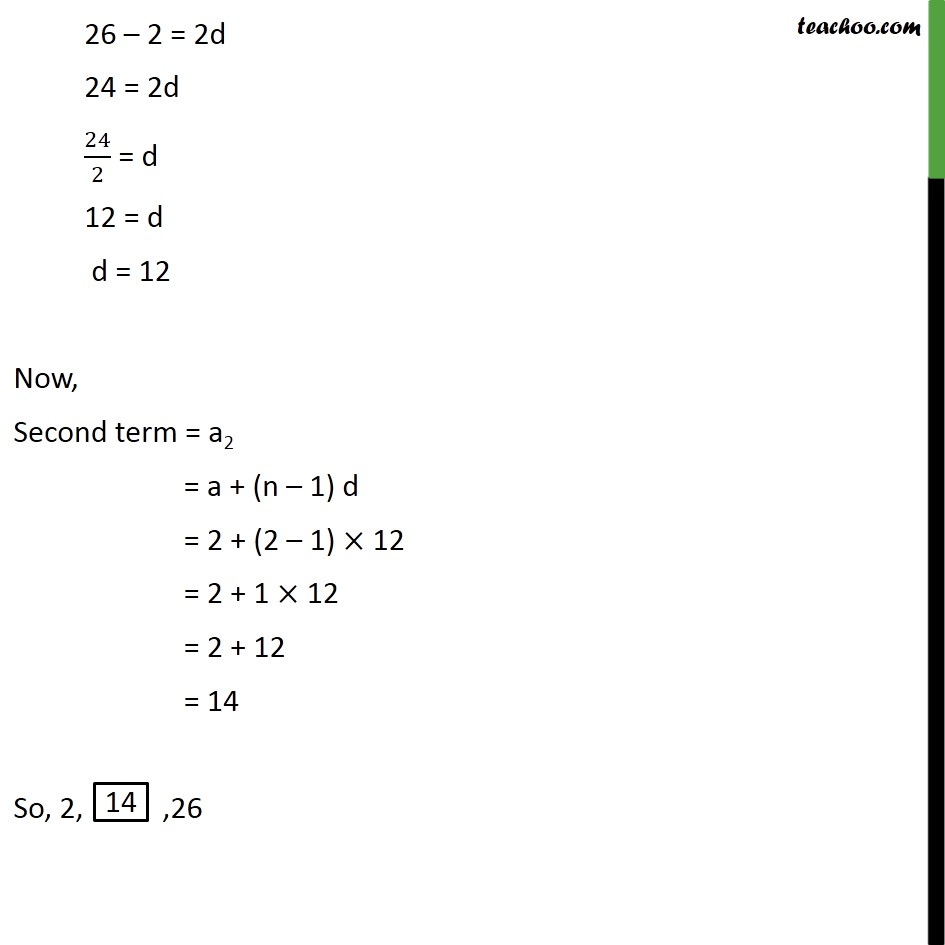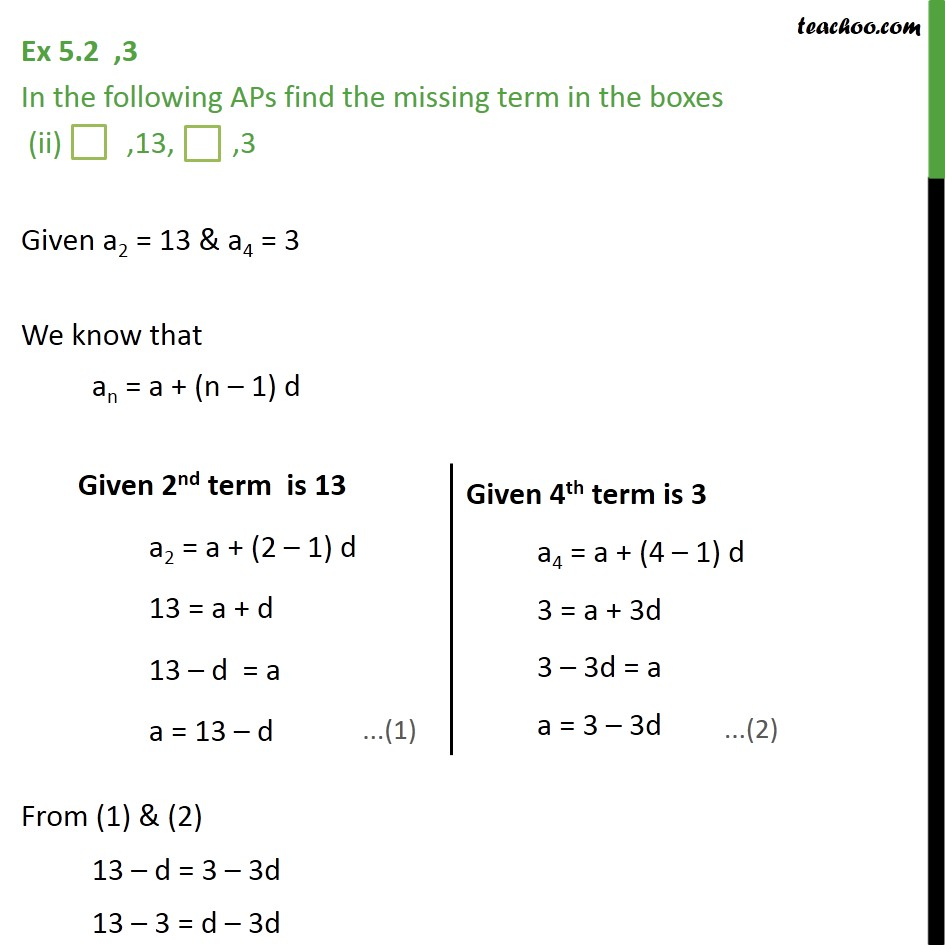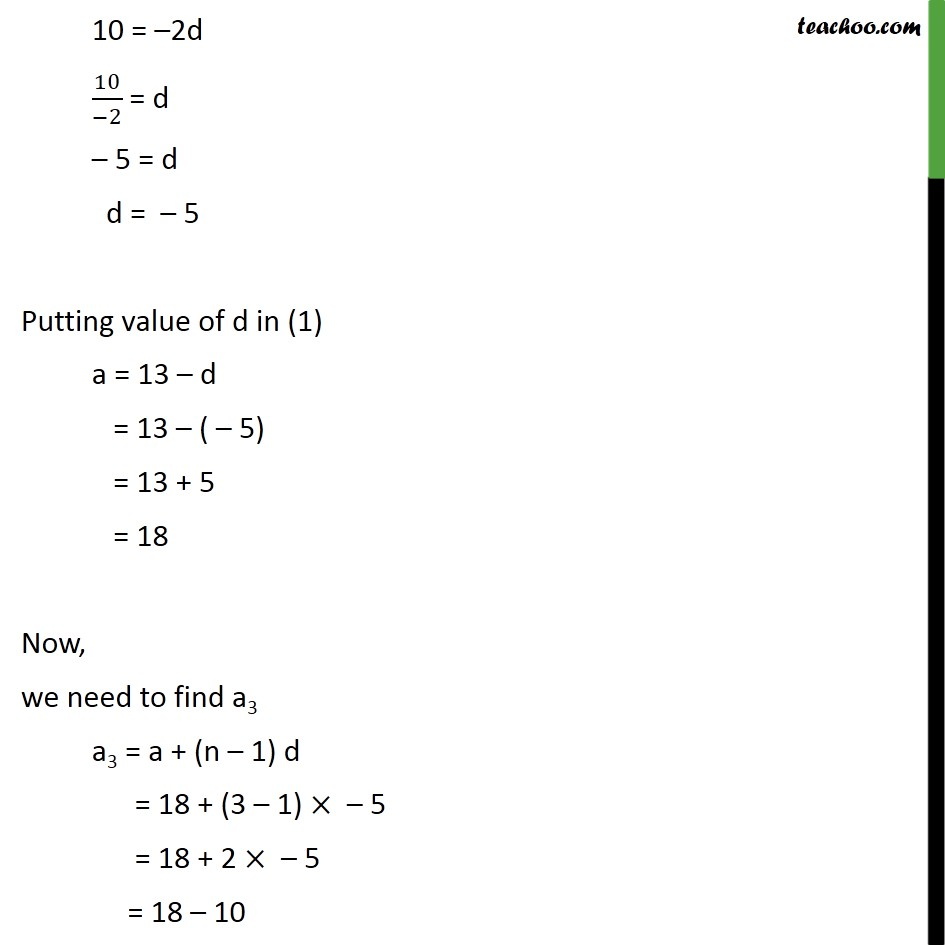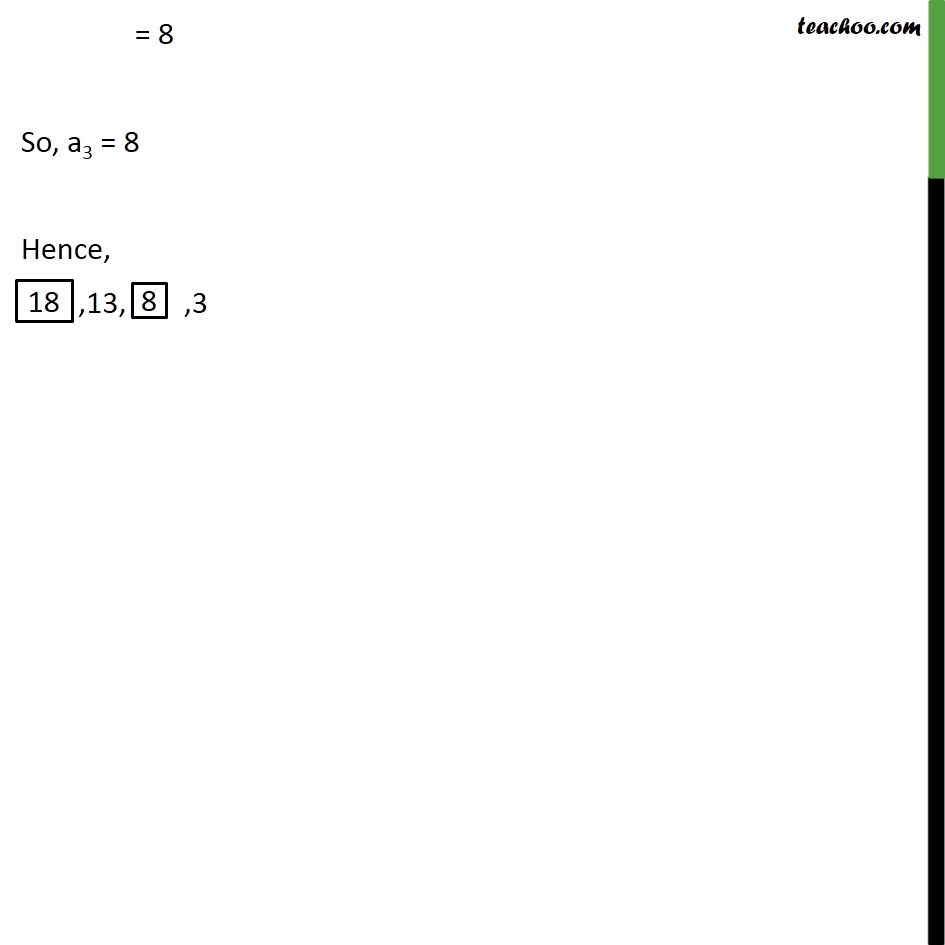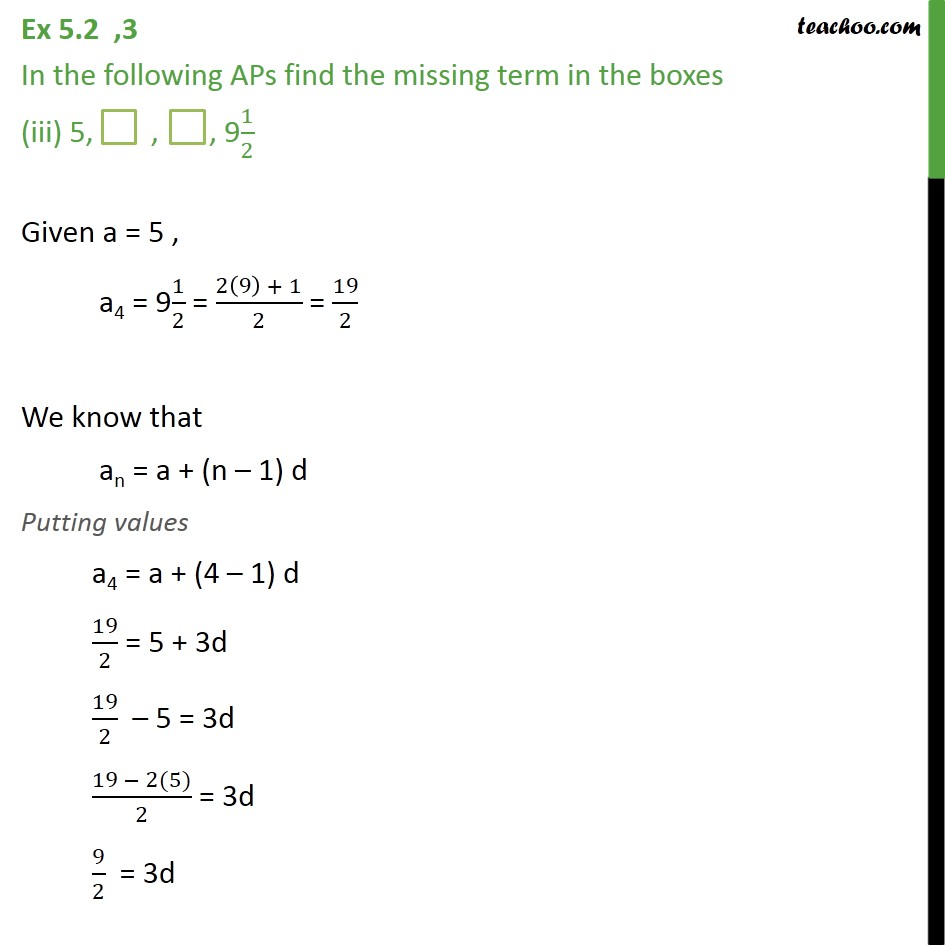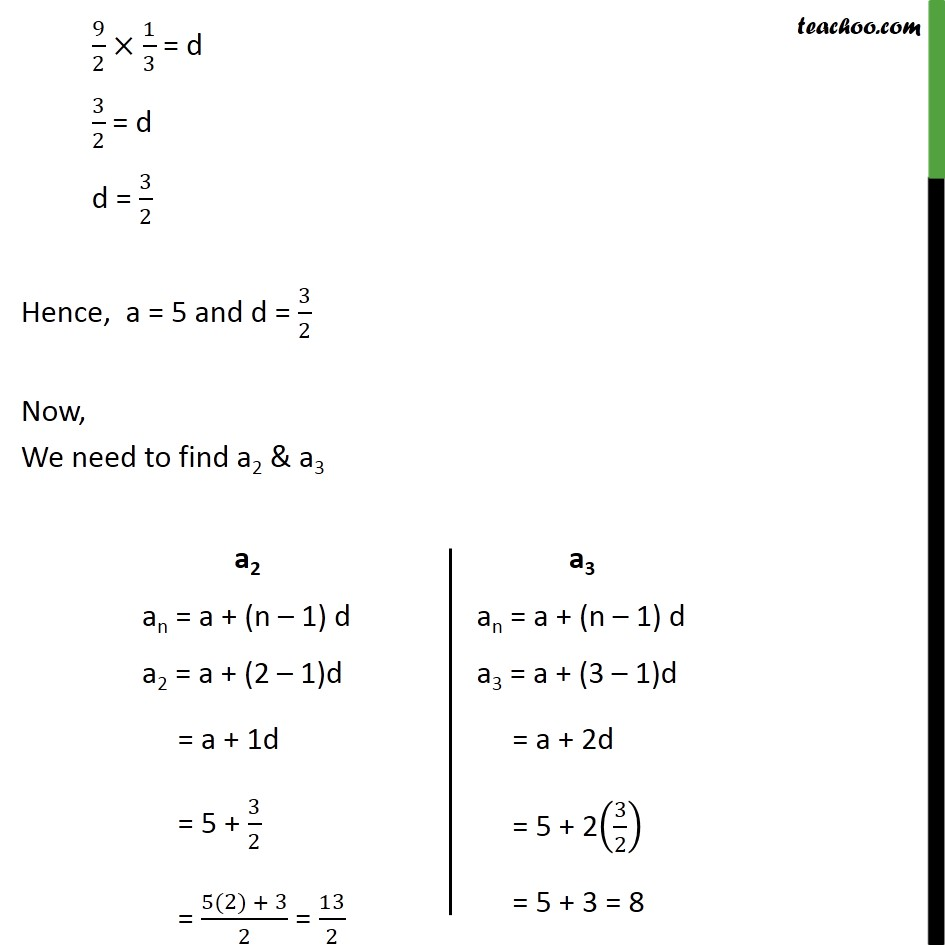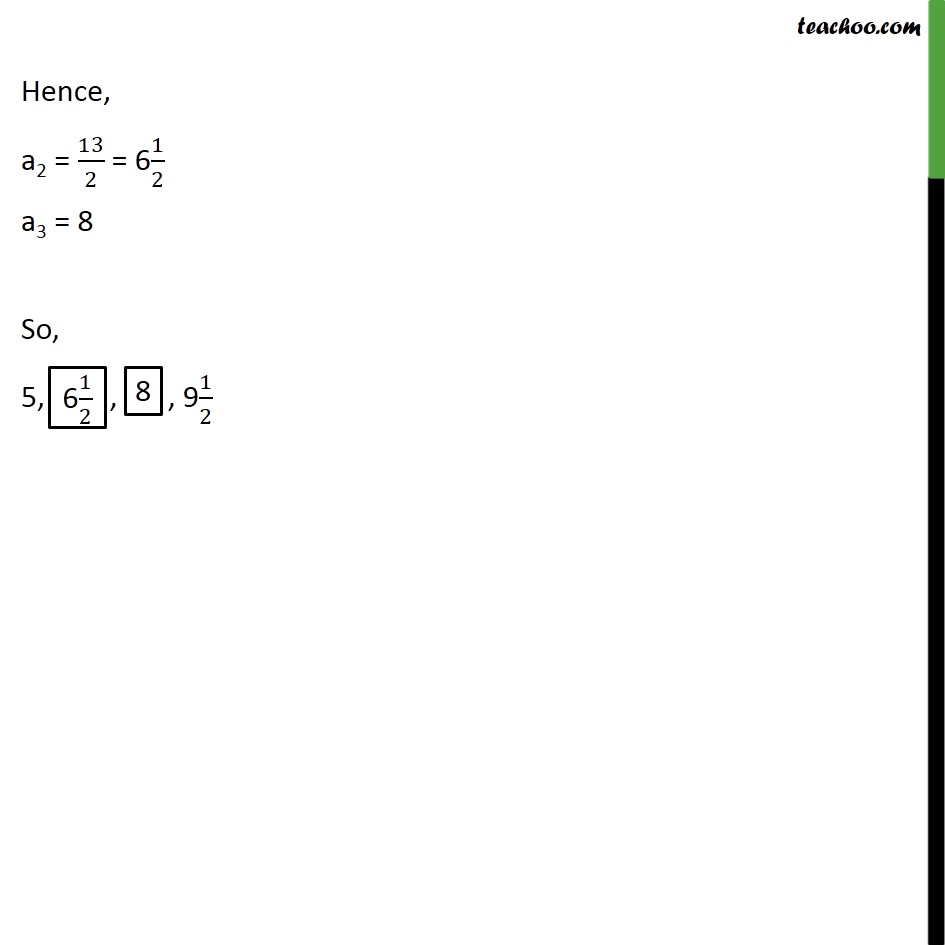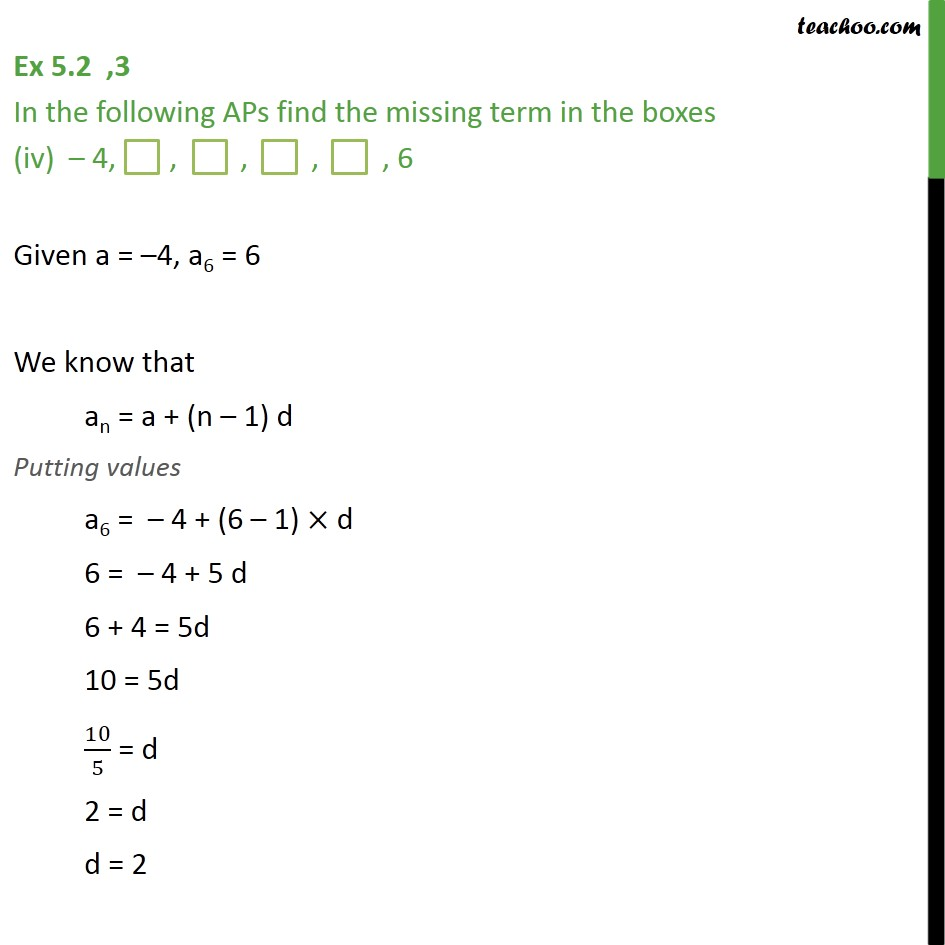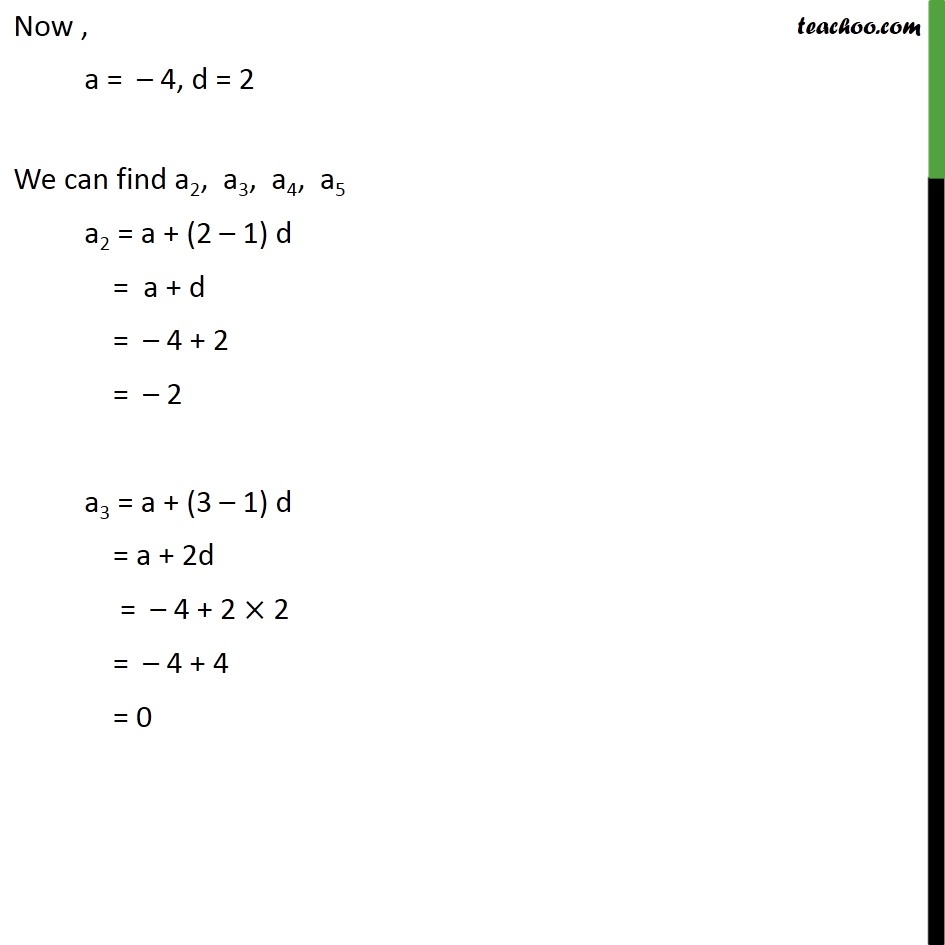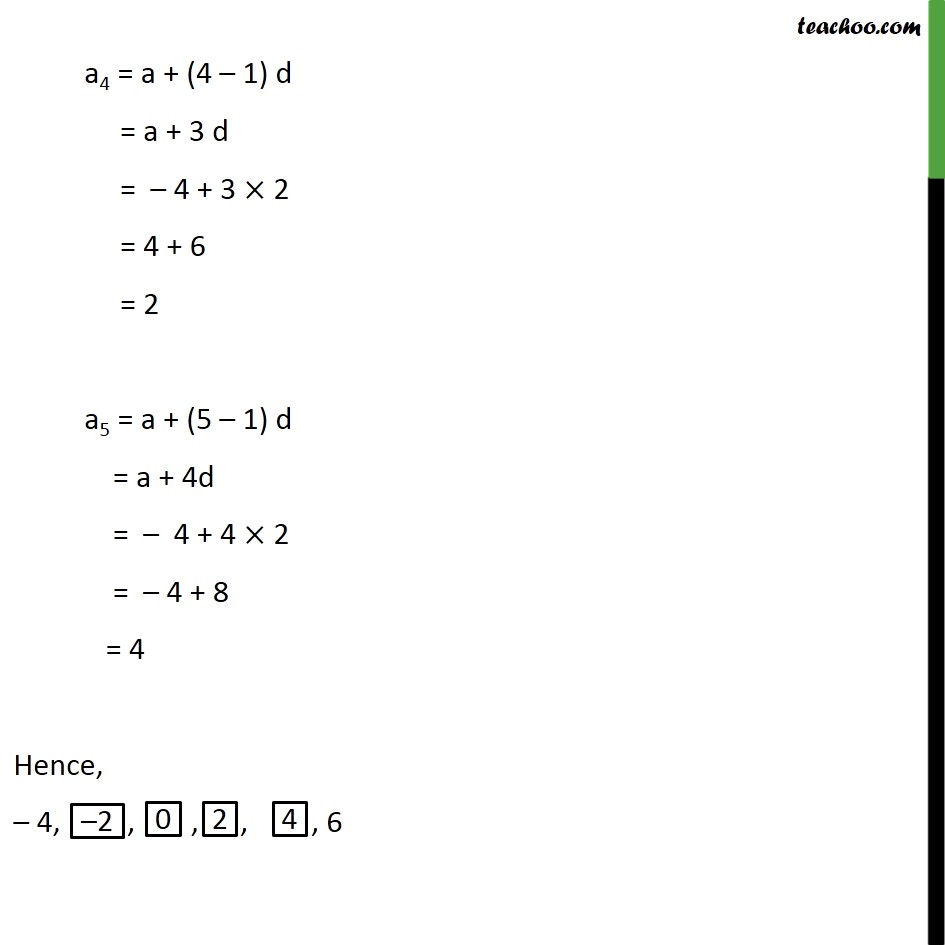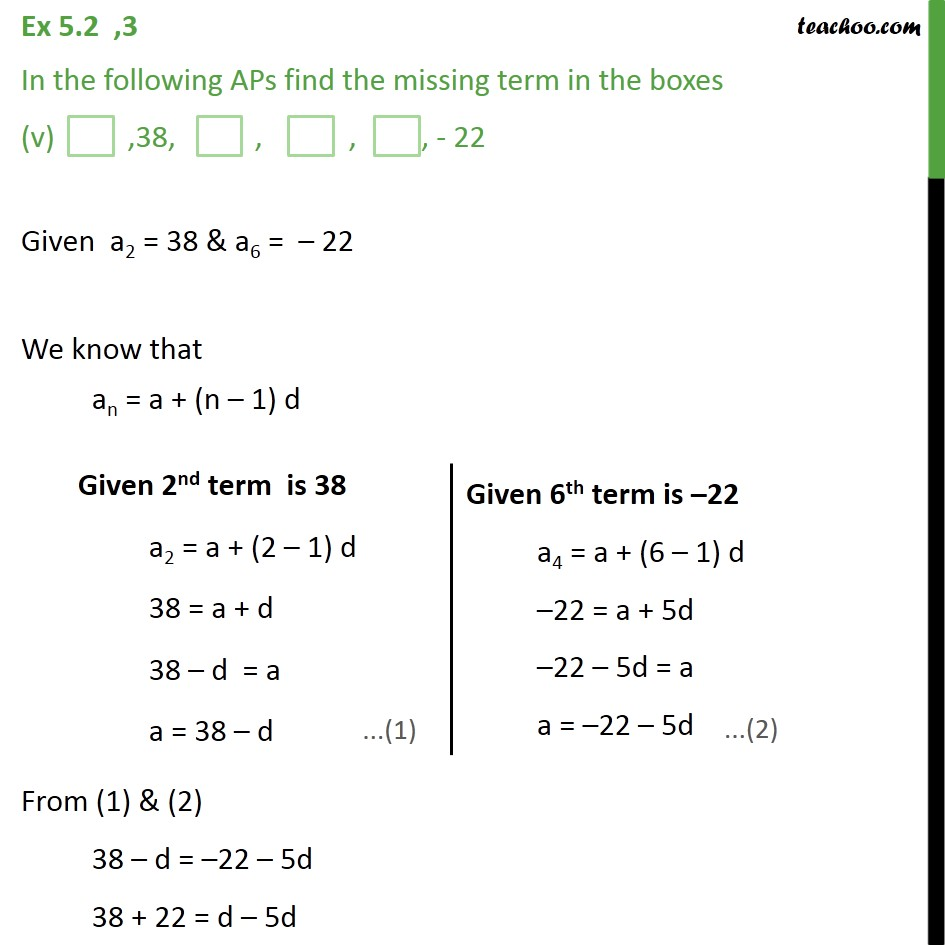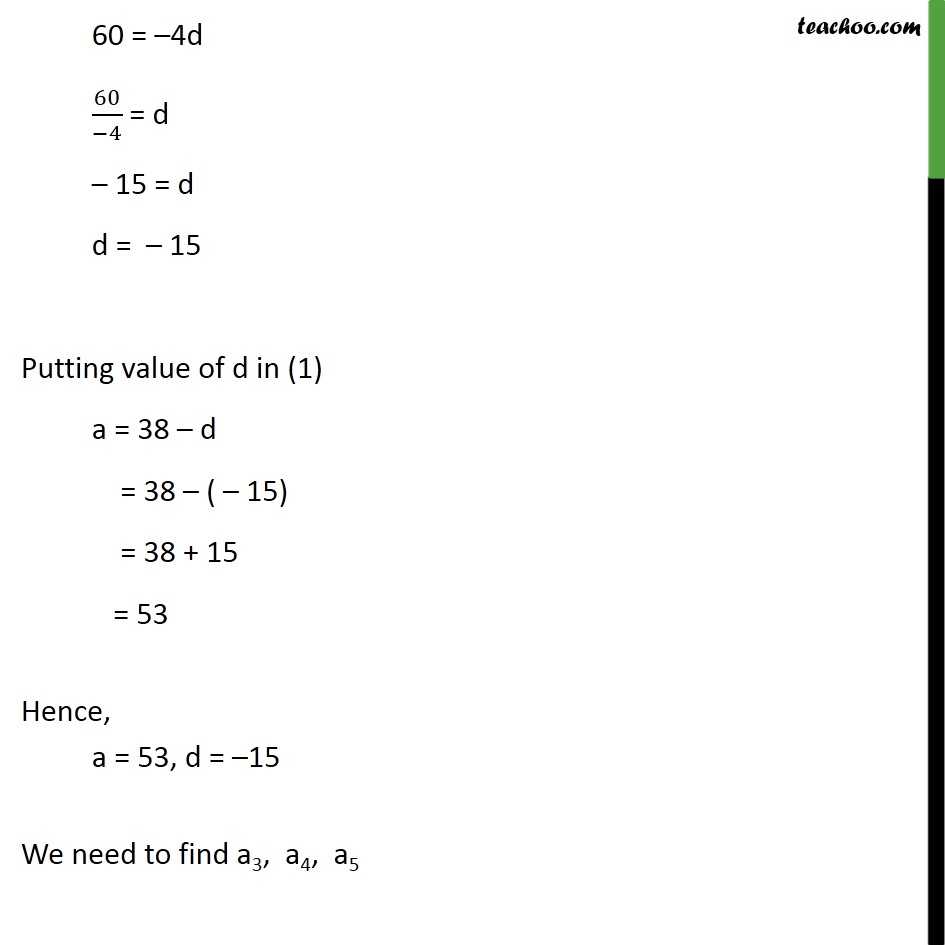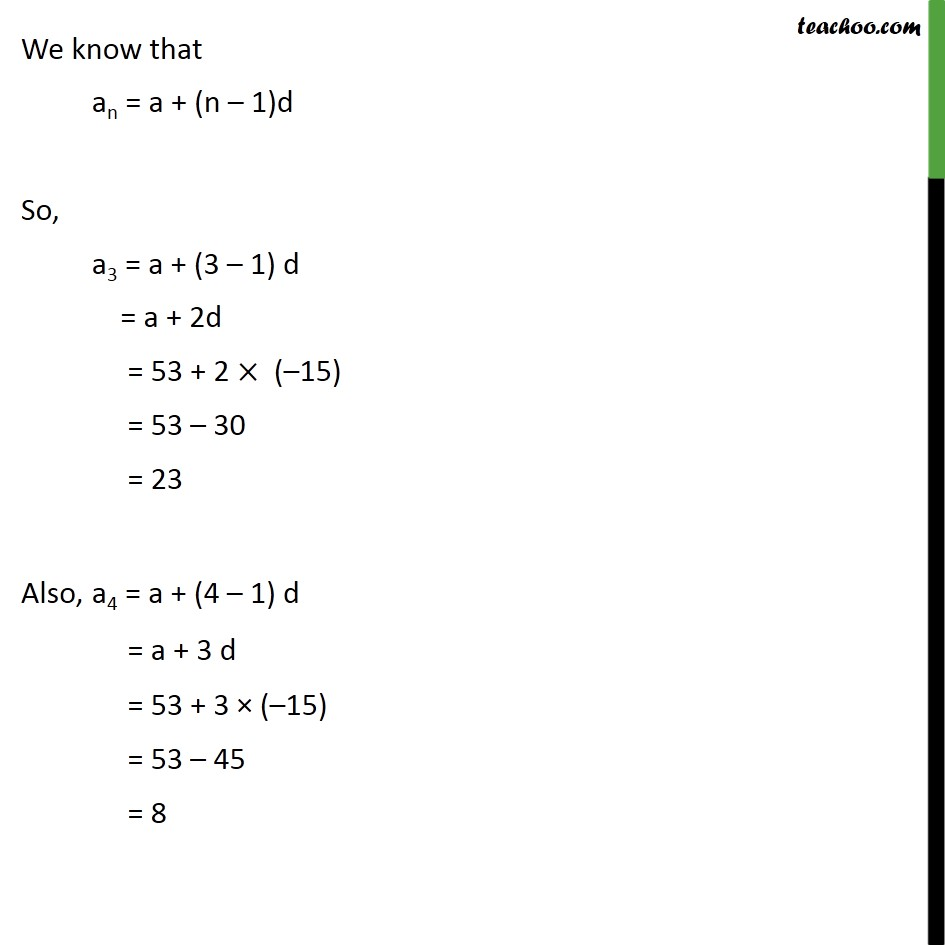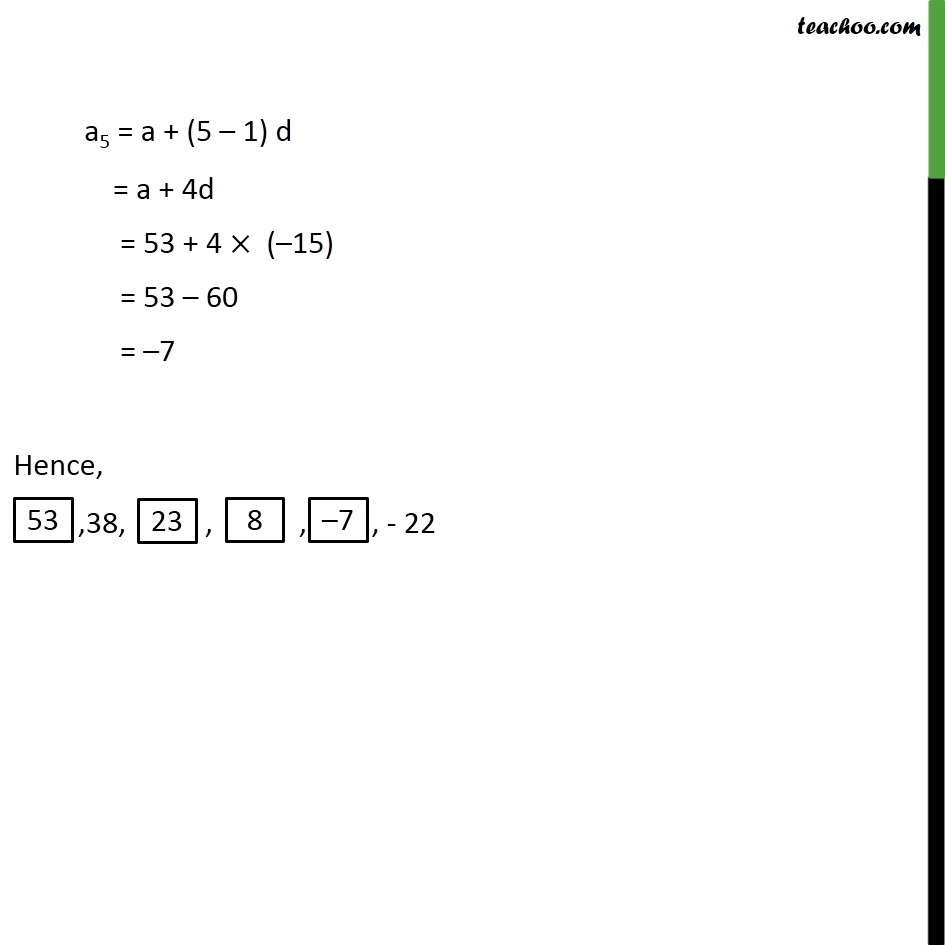1. Chapter 5 Class 10 Arithmetic Progressions
2. Serial order wise
3. Ex 5.2

Transcript

Ex 5.2 ,3 (Method 1) In the following APs find the missing term in the boxes (i) 2, ,26 Let 2nd term = x So, AP is 2, x, 26 Since it is an AP, common difference is same ∴ Second term – First term = Third term – Second term x – 2 = 26 – x x + x = 2 + 26 2x = 28 x = 28/2 x = 14 So, 2, ,26 Ex 5.2 ,3 (Method 2) In the following APs find the missing term in the boxes (i) 2, ,26 Given a = 2 a3 = 26 We need to find a2 We know that an = a + (n – 1) d Here , a3 = 26 , a = 2 ,n = 3 First we find d Putting values in formula an = a + (n – 1) d 26 = 2 + (3 – 1)d 26 = 2 + 2d 26 – 2 = 2d 24 = 2d 24/2 = d 12 = d d = 12 Now, Second term = a2 = a + (n – 1) d = 2 + (2 – 1) × 12 = 2 + 1 × 12 = 2 + 12 = 14 So, 2, ,26 Ex 5.2 ,3 In the following APs find the missing term in the boxes (ii) ,13, ,3 Given a2 = 13 & a4 = 3 We know that an = a + (n – 1) d From (1) & (2) 13 – d = 3 – 3d 13 – 3 = d – 3d 10 = –2d 10/(−2) = d – 5 = d d = – 5 Putting value of d in (1) a = 13 – d = 13 – ( – 5) = 13 + 5 = 18 Now, we need to find a3 a3 = a + (n – 1) d = 18 + (3 – 1) × – 5 = 18 + 2 × – 5 = 18 – 10 = 8 So, a3 = 8 Hence, ,13, ,3 Ex 5.2 ,3 In the following APs find the missing term in the boxes (iii) 5, , , 91/2 Given a = 5 , a4 = 91/2 = (2(9) + 1)/2 = 19/2 We know that an = a + (n – 1) d Putting values a4 = a + (4 – 1) d 19/2 = 5 + 3d 19/2 – 5 = 3d (19 − 2(5))/2 = 3d 9/2 = 3d 9/2 × 1/3 = d 3/2 = d d = 3/2 Hence, a = 5 and d = 3/2 Now, We need to find a2 & a3 Hence, a2 = 13/2 = 61/2 a3 = 8 So, 5, , , 91/2 Ex 5.2 ,3 In the following APs find the missing term in the boxes (iv) – 4, , , , , 6 Given a = –4, a6 = 6 We know that an = a + (n – 1) d Putting values a6 = – 4 + (6 – 1) × d 6 = – 4 + 5 d 6 + 4 = 5d 10 = 5d 10/5 = d 2 = d d = 2 Now , a = – 4, d = 2 We can find a2, a3, a4, a5 a2 = a + (2 – 1) d = a + d = – 4 + 2 = – 2 a3 = a + (3 – 1) d = a + 2d = – 4 + 2 × 2 = – 4 + 4 = 0 a4 = a + (4 – 1) d = a + 3 d = – 4 + 3 × 2 = 4 + 6 = 2 a5 = a + (5 – 1) d = a + 4d = – 4 + 4 × 2 = – 4 + 8 = 4 Hence, – 4, , , , , 6 Ex 5.2 ,3 In the following APs find the missing term in the boxes (v) ,38, , , , - 22 Given a2 = 38 & a6 = – 22 We know that an = a + (n – 1) d From (1) & (2) 38 – d = –22 – 5d 38 + 22 = d – 5d 60 = –4d 60/(−4) = d – 15 = d d = – 15 Putting value of d in (1) a = 38 – d = 38 – ( – 15) = 38 + 15 = 53 Hence, a = 53, d = –15 We need to find a3, a4, a5 We know that an = a + (n – 1)d So, a3 = a + (3 – 1) d = a + 2d = 53 + 2 × (–15) = 53 – 30 = 23 Also, a4 = a + (4 – 1) d = a + 3 d = 53 + 3 × (–15) = 53 – 45 = 8 a5 = a + (5 – 1) d = a + 4d = 53 + 4 × (–15) = 53 – 60 = –7 Hence, ,38, , , , - 22

Ex 5.2